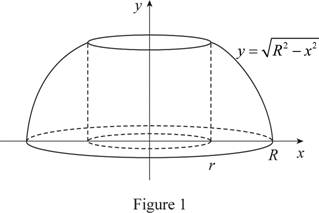# the more wood content of the ring from two rings.### Single Variable Calculus: Concepts...

4th Edition
James Stewart
Publisher: Cengage Learning
ISBN: 9781337687805### Single Variable Calculus: Concepts...

4th Edition
James Stewart
Publisher: Cengage Learning
ISBN: 9781337687805

#### Solutions

Chapter 6.3, Problem 38E

a)

To determine

## To find: the more wood content of the ring from two rings.

Expert Solution

The both napkin rings are having a same quantity of wood.

### Explanation of Solution

The volume only depends up on the height of the napkin ring.

Hence, the both napkin rings are having same quantity of wood.

b)

To determine

### To check: The part (a) answer by computing the volume of a napkin ring using cylindrical shells.

Expert Solution

The volume of a napkin ring is πh36_

### Explanation of Solution

Given:

The height of the napkin ring is h.

The radius of the napkin ring is r.

The radius of the sphere is R.

Calculation:

Consider the equation of napkin ring as follows:

y=R2x2 (1)

The region lies between x=r and x=R.

Sketch the solid region as shown below in Figure 1.Refer Figure 1

Calculate the volume using the method of cylindrical shell as follows.

V=2×ab2πx[f(x)]dx (2)

Substitute r for a, R for b, and R2x2 for [f(x)] in equation (2).

V=2rR2πxR2x2dx=2πrR2xR2x2dx (3)

Consider u=R2x2 (4)

Differentiate both sides of the equation.

du=2xdxdu=2xdx

Calculate the lower limit value of u using equation (4).

Substitute r for x in equation (4).

u=R2r2

Calculate the upper limit value of u using equation (4).

Substitute R for x in equation (4).

u=R2R2=0

Apply lower and upper limits for u in equation (3).

Substitute u for (R2x2) and (du) for 2xdx dx in equation (3).

V=2πR2+r20u(du)=2πR2+r20u12du (5)

Integrate equation (5).

V=2π[u12+112+1]R2+r20=2π[u3232]R2+r20=2π[2(0)3232(R2+r2)323]=43π(R2+r2)32 (6)

Consider R2+r2=(h2)2

Substitute (h2)2 for R2+r2 in equation (6).

V=43π(h2)2×32=424πh3=16πh3

Hence, the volume of a napkin ring is πh36_.

### Have a homework question?

Subscribe to bartleby learn! Ask subject matter experts 30 homework questions each month. Plus, you’ll have access to millions of step-by-step textbook answers!